# Fractions and Decimals on a Number Line: A Study Guide

## Whole Numbers

Whole numbers, also called integers or counting numbers, are the numbers we use to show complete quantities. Whole numbers are written without a decimal or fraction. For example:

• I read one book this summer.
• There are five cats in my Grandma’s house.
• I am in debt. I have -\$10 because I spent \$10 more than I had available.

## Fractions

Fractions are numbers used to express a part of a whole. Fractions are written as a ratio of the number of parts to the whole. The parts are always of equal value.

• I ate ½ of the pizza. (The pizza was divided into two equal parts. I ate one of those two parts.)
• Only ¾ of the class went on the field trip. (If the class were divided into four equal groups, three out of four groups went on the trip.)
• I wasn’t going to buy a whole pineapple, so I ordered 1/3 of a pineapple.

## Decimals

Decimals are used just like fractions—to show a part of a whole. Decimals are written using the same symbols as whole numbers but are distinguished by a dividing period, called a decimal point. The decimal places, those to the right of the period, also have place value just like whole numbers.

• .1 is one tenth. Another way of talking about 1 out of 10 parts.
• .15 is one tenth and five hundredths—or 15 out of 100 parts.
• The movie is 1.5 hours long. (One complete hour and five out of ten parts of an hour. )
• I spend \$4.56 on a birthday card for my Uncle Tom. (Four whole dollars and 56 out of 100 parts of a dollar–56 pennies.)

## Number Line

A number line is constructed of one long horizontal line and several vertical lines. The vertical lines, sometimes called ticks or marks, are used to divide the number line into equal sections. (i.e., equal parts) Labels on the ticks tell you what each one means.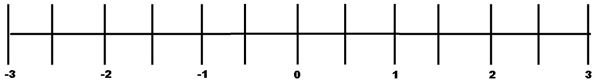In this number line, there are three negative and three positive integers. (i.e., -3, -2, -1, 1, 2, 3) Between each number there is an unlabeled tick. Since they are halfway between one number and the next, we can deduce that these unlabeled marks represent half values. (i.e., 1/2 or .5)

On a number line, fractions and decimals are represented the same way–as parts of a whole. Fractions and decimals of equal value will be shown the same way. (e.g., 1/4 will look the same was .25)

## Example 1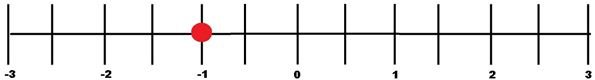Look at the red dot on the number line above. It falls exactly on the line marked "-1." This is a negative integer so we know that we are talking about a negative value. In this case, having 1 unit less than zero. (Since the graph is not labeled with a title this number could represent many things: a test score, money owed, yards in a football game.)

## Example 2The graph (above) has a red dot on an unmarked tick halfway between 0 and 1. Since we know that every other line has a value of one unit, we can deduce that every tick has a value of one half. To find the value of the dot you can reference the line before or ahead of the indicated mark.

• 0 plus one half is one half (i.e., 1/2 or .5) or
• 1 minus one half is one half

## Example 3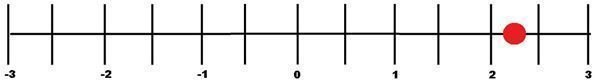Since the dot is not on a tick we will only be able to estimate the value of the dot. This one is a bit tricky so let's take it one step at a time.

1. Identify the ticks closest to the dot. The dot is between 2 and 2.5. You can see that the whole number 2 has already been passed, so the value of the dot is 2 plus an unknown fraction. Steps 2 and 3 will help you find the value of this part.
2. Estimate the position of the dot. The dot is halfway between 2 and 2.5.
3. Figure out the value of 1/2 of 1/2, .5 of .5. Multiply the fractions/decimals to find the value of the dot's position. (1/4 or .25) Alternately, use another visual aid to help you figure this out. Draw a circle. Divide the circle in half and divide the circle in half again. The value of one section is–one quarter!
4. Add the value of this fraction/decimal with the whole number value of the dot. (2 + .25 or 2 + 1/4)

The sum is 2.25 or 2 1/4

## Practice Problems

Can you figure out the value of the green dots for each of these graphs? (The answer key is after the Resources)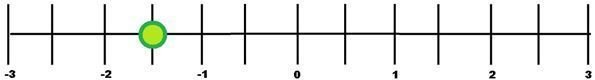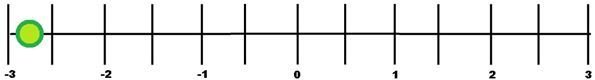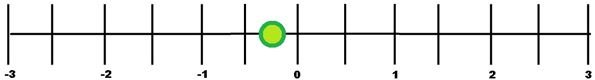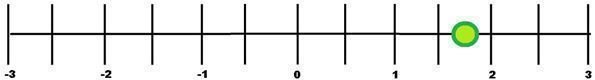Resources

• Learning Wave: Number Line (https://www.learningwave.com/chapters/integers/numline.html)
• Maths Dictionary For Kids (https://www.amathsdictionaryforkids.com/dictionary.html)
• Helping With Math: Number Lines (https://www.helpingwithmath.com/resources/oth_number_lines.htm)
• Fun Brain: Number Line Game (https://www.funbrain.com/linejump/index.html)
• Math Is Fun: Number Line (https://www.mathsisfun.com/number-line.html)

Photo Credit

“Number Line Series” by Sylvia Cini. Creative Commons.